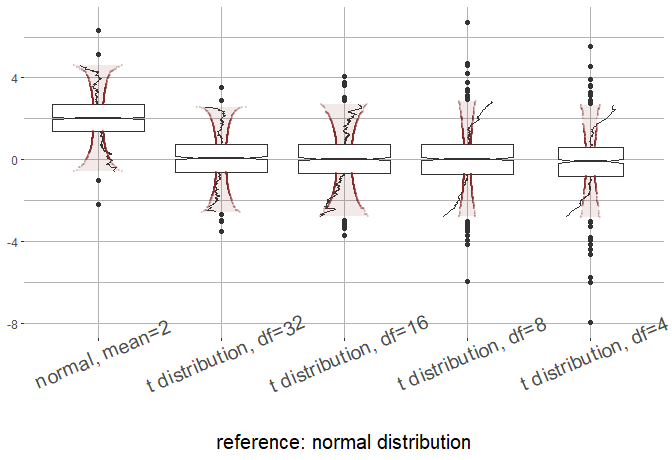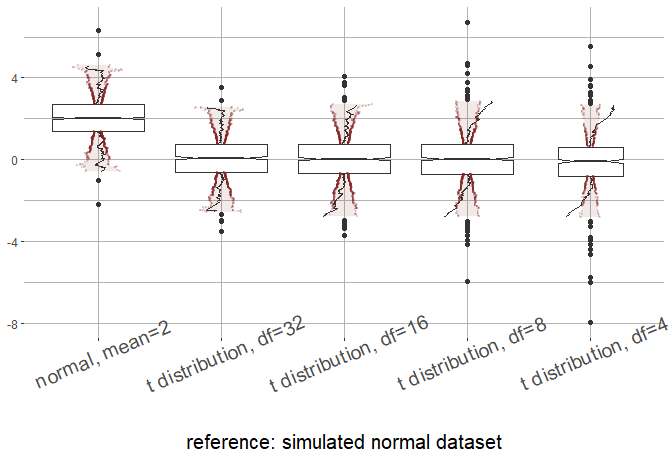# Basic usage for the qqboxplot package

This vignette introduces some basic usage of the R package qqboxplot. The figures below are reproductions of the figures found in “The q-q boxplot” (citation coming soon). We first start by reproducing figures that use the q-q boxplot. The other figures used for comparison in the paper follow after that.

## qqboxplot

First load the ‘qqboxplot’ package and packages from the ‘tidyverse’.

library(dplyr)
library(ggplot2)
library(qqboxplot)

The following figure compares simulated t-distributions (and one simulated normal distribution) against a theoretical normal distribution. simulated_data contains to columns, “y” and “group”.
“group” specifies the distribution the data (“y”) comes from. Note in this figure that reference_dist = “norm” is chosen to specify that the normal distribution should be the reference distribution.

simulated_data %>%
ggplot(aes(factor(group, levels=c("normal, mean=2", "t distribution, df=32", "t distribution, df=16", "t distribution, df=8", "t distribution, df=4")), y=y)) +
geom_qqboxplot(notch=TRUE, varwidth = TRUE, reference_dist="norm") +
xlab("reference: normal distribution") +
ylab(NULL) +
guides(color=FALSE) +
theme(axis.text.x = element_text(angle = 23, size = 15), axis.title.y = element_text(size=15),
axis.title.x = element_text(size=15),
panel.border = element_blank(), panel.background = element_rect(fill="white"),
panel.grid = element_line(colour = "grey70"))
#> Warning: guides(<scale> = FALSE) is deprecated. Please use guides(<scale> =
#> "none") instead.simulated data was created by running the following code:

tibble(y=c(rnorm(1000, mean=2), rt(1000, 16), rt(500, 4),
rt(1000, 8), rt(1000, 32)),
group=c(rep("normal, mean=2", 1000),
rep("t distribution, df=16", 1000),
rep("t distribution, df=4", 500),
rep("t distribution, df=8", 1000),
rep("t distribution, df=32", 1000)))

The following figure shows the same data as the previous figure, but compared against a simulated normal distribution, with mean=5 and variance=1. Note that the reference dataset comparison_dataset is a separate vector and is not contained in the dataset simulated_data.

simulated_data %>%
ggplot(aes(factor(group, levels=c("normal, mean=2", "t distribution, df=32", "t distribution, df=16", "t distribution, df=8", "t distribution, df=4")), y=y)) +
geom_qqboxplot(notch=TRUE, varwidth = TRUE, compdata=comparison_dataset) +
xlab("reference: simulated normal dataset") +
ylab(NULL) +
theme(axis.text.x = element_text(angle = 23, size = 15), axis.title.y = element_text(size=15),
axis.title.x = element_text(size=15),
panel.border = element_blank(), panel.background = element_rect(fill="white"),
panel.grid = element_line(colour = "grey70"))The vector comparison_dataset was simulated as follows

rnorm(1000, 5)